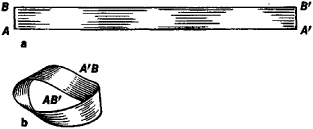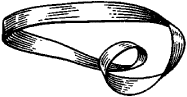# Möbius Band

Also found in: Dictionary, Thesaurus.

## Möbius band

[′mər·bē·əs ‚band]
(mathematics)
The nonorientable surface obtained from a rectangular strip by twisting it once and then gluing the two ends. Also known as Möbius strip.

## Möbius Band

(also Möbius strip), a surface obtained by pasting together two opposite sides AB and A’B’ of a rectangle ABB’A’ (see Figure 1, a) such that the points A and B coincide with the points B’ and A’, respectively (Figure 1, b). The Mobius strip was studied independently (between 1858 and 1865) by the German mathematicians A. Mobius and J. Listing as the first example of a one-sided surface.Figure 1. Construction of MÖbius band: (a) starting rectangle, (b) Möbius band

If we move along a MÖbius strip (or along any other one-sided surface) without crossing its edge, then, in distinction to the case of two-sided surfaces (such as a sphere or cylinder), it is possible to return to the initial point, on the “opposite side.” This is due to the fact that the MÖbius band is not orientable. Specifically, if we draw a small oriented circle on the band and move it along the band without crossing the edge, then it is possible to arrive at the initial position with reversed orientation of the circle. The MÖbius band is bounded by only a single closed curve. Therefore, if a MÖbius band is cut along the middle, it remains one piece and becomes a surface homeomorphic to the surface of a cylinder, differing from the latter only in that it twists twice about itself (Figure 2).Figure 2. Surface obtained when MÖbius band is cut along center line

From the topological point of view, the MÖbius band is a nonorientable surface with zero Euler characteristic bounded by a single closed circle.

Site: Follow: Share:
Open / Close